# AP Statistics Unit 1 Practice Test: Exploring One-Variable Data

Test 1 for AP Stats.

Congratulations - you have completed .

You scored %%SCORE%% out of %%TOTAL%%.

Your performance has been rated as %%RATING%%

 Question 1

### On a school survey; your age, gender, height, GPA, and highest level of education are recorded.  The number and type of variables you recorded is:

 A 5 quantitative, 0 categorical B 3 quantitative, 2 categorical C 2 quantitative, 2 categorical D 2 quantitative, 3 categorical
Question 1 Explanation:
In this survey, 5 variables are measured: age, gender, height, GPA and highest level of education. Quantitative variables are variables that take on numerical values for a measured or counted quantity. The 3 quantitative variables are age (measured in number of years), height (measured in inches or centimeters), and GPA (measured on a 4-point scale). A categorical variable takes on values that are category names or group labels. The 2 categorical variables are gender and highest level of education.
 Question 2

### The boxplots summarize the distribution of family size (number of kids in the family) amongst how much education the mothers have.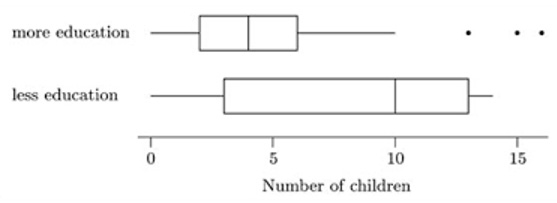4. ### There are no outliers amongst those with more education

 A I only B I and II C II and III D II, III, and IV
Question 2 Explanation:
I. Incorrect. The range of more education is bigger than the range of less education

II. Correct. The lowest number of children for both groups is 0

III. Correct. The distribution of more education is roughly symmetric with outliers. The less education distribution is skewed to the right since the median is pulled to the direction of the right.

IV. Incorrect. There are three outliers represented by dots in the more education group.
 Question 3

4. ### Age of competitors in a race

 A I B II, III, and IV C I and IV D None of the above
Question 3 Explanation:
I (number of students entering a race) is the only discrete variable. The number of students can’t be divided into smaller parts, but time, age, and height can be divided into smaller and smaller fractions of units.
 Question 4

### The boxplot shows the data gathered based on 100 purchases at a craft vendor’s table. Based on the results, half of all purchases fall between which two price points?A \$2-\$5 B \$2-\$2 C \$5-\$20 D \$5-\$12
Question 4 Explanation:
A box plot shows the quartiles of a data set, with each segment (whisker-to-box or each half of the box) representing one-quarter of the data. Therefore the segment $5-$12, which spans both parts of the box, represents 50% of the data.
 Question 5

### The histogram below shows the age of 30 employees of a company.  Which of the following statements is correct?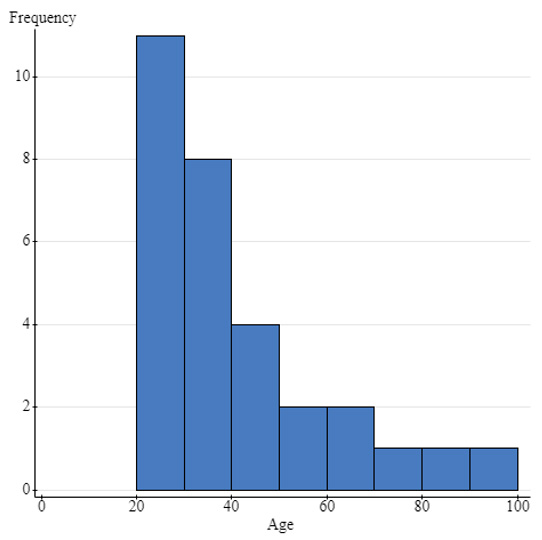A The distribution is symmetric. B The distribution is skewed to the right. C The distribution is skewed to the left. D We are not able to determine the type of distribution
Question 5 Explanation:
Most of the data appears on the left hand side of the histogram. There is no “center” value and there are a few data points pulling the data to be skewed to the right.
 Question 6

### The histogram below shows the age of 30 employees of a company.  Determine the median age range.A Between 40 and 50 B Between 50 and 60 C Between 20 and 30 D Between 30 and 40
Question 6 Explanation:
Since there are 30 data points, the median would be the 15th data point when they are in order from least to greatest. The first column represents the first 11 data points in order, the second column represents the next 8 data points. The 15th data point is in column two which is the range 30 to 40. The median age range is between 30 and 40.
 Question 7

### At a burger stand, 250 people were surveyed on whether they preferred eating their burgers with cheese or no cheese.  Of the 250 people surveyed, 100 were adults and 150 were children.  Of the responses, 160 people said they prefer cheese and 100 of those were children. For those surveyed, there was no association between age and preference of cheese or not.  Which of the following tables shows the distribution of responses?

 A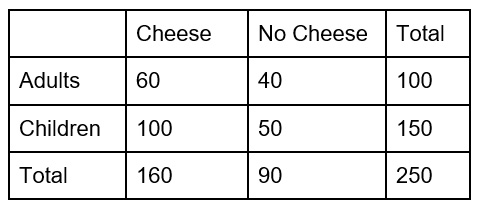B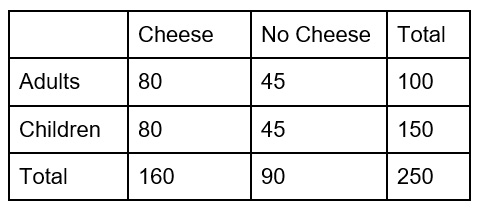C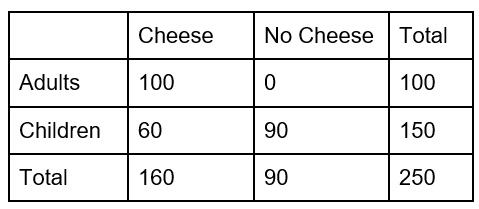DQuestion 7 Explanation:
First fill in the numbers that we know.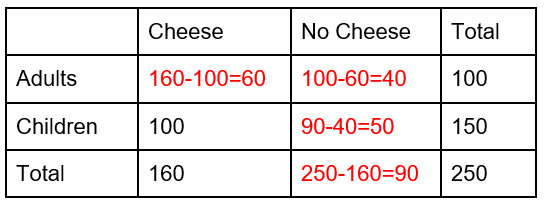For each column and row, the cells have to add to the total. We can use addition and subtraction to find what is in the missing boxes.
 Question 8

### Below is the starting salary for 150 new employees at a company.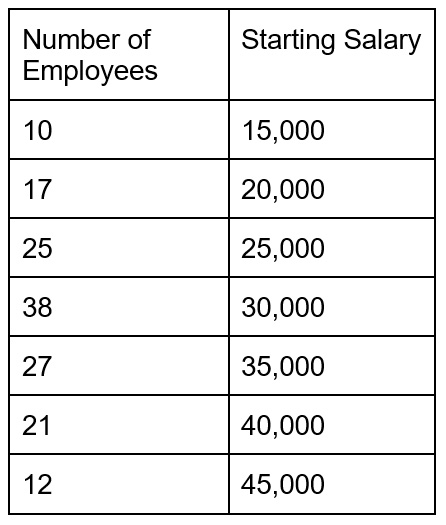### What is the mean starting salary?

 A $30,000 B$30,533 C $32,500 D 35,000 Question 8 Explanation: In order to find the mean, add up all of the data points and then divide by the total which is 150. When looking at the table, note that 10 people had a salary of \$15,000.

$\dfrac{10(15,000,)+17(20,000)+25(25,000)+38(30,000)+27(35,000)+21(40,000)+12(45,000)}{150} = 30,533.33$

The mean starting salary is $30,533  Question 9 ### Consider the dot plot below.### What is the 5 number summary of the distribution above?  A 1,7,9,10,11 B 1,7,10,11,11 C 1,8,9,10,11 D 1,9,10,10,11 Question 9 Explanation: The five number summary consists of the: minimum, quartile 1, median, quartile 3, and the maximum. The minimum value is 1. The maximum value is 11. The median is the point exactly in the middle which is 9. The quartile 1 is the median of the first half of the data which is 7. The quartile 3 is the median of the second half of the data which is 10.  Question 10 ### The results of a classroom set of test scores is below. Which option displays the correct box plot for the data? ### 60, 62, 64, 64, 72, 75, 78, 80, 80, 81, 81, 82, 85, 89, 92, 94, 97  A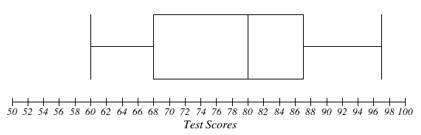B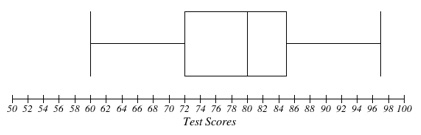C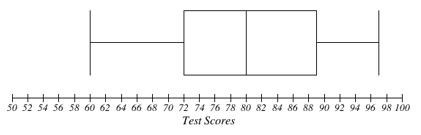D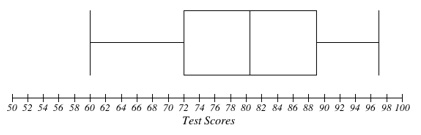Question 10 Explanation: Answer (a) is correct. The median of the data set is the middle number, 80. Quartile one is the middle of the bottom half, 68, which is the median of 64 and 72. Quartile three is the middle of the top half, 87, which is the median of 85 and 89.  Question 11 ### The height of a selected sample of boys in the United States is normally distributed with a mean of 64 inches and a standard deviation of 0.5 inches. The height of a selected sample of girls is normally distributed with a mean of 62 inches and a standard deviation of 0.4 inches. Approximately what is the height of a boy with the same z-score as a girl with a height of 63 inches?  A 62.75 inches B 65.25 inches C 63.25 inches D 64.75 inches Question 11 Explanation: The formula for z-score is$\dfrac{(x-μ)}{σ}$, where$x$is the observed value,$µ$is the mean, and σ is the standard deviation.$\dfrac{(63-62)}{0.4} = \dfrac{(x-64)}{0.5}$, giving an$x$value of$65.25\$.
 Question 12

4. ### They can display frequencies or relative frequencies

 A II, III, and IV B II and III C I and IV D II and IV
Question 12 Explanation:
A bar graph is used to display categorical data. It is often mistaken for a histogram which displays qualitative data and does not have space between the bars. It can display frequencies (counts) or relative frequencies (proportions). The order of the categories does not matter and every bar represents a different category.
Once you are finished, click the button below. Any items you have not completed will be marked incorrect.
There are 12 questions to complete.
 ← List →